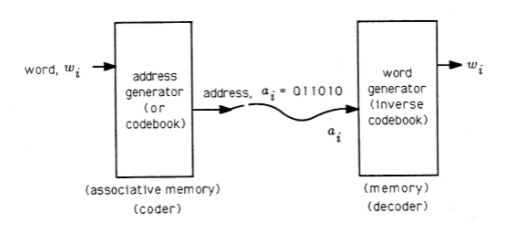# 7.1: Introduction

$$\newcommand{\vecs}{\overset { \rightharpoonup} {\mathbf{#1}} }$$ $$\newcommand{\vecd}{\overset{-\!-\!\rightharpoonup}{\vphantom{a}\smash {#1}}}$$$$\newcommand{\id}{\mathrm{id}}$$ $$\newcommand{\Span}{\mathrm{span}}$$ $$\newcommand{\kernel}{\mathrm{null}\,}$$ $$\newcommand{\range}{\mathrm{range}\,}$$ $$\newcommand{\RealPart}{\mathrm{Re}}$$ $$\newcommand{\ImaginaryPart}{\mathrm{Im}}$$ $$\newcommand{\Argument}{\mathrm{Arg}}$$ $$\newcommand{\norm}{\| #1 \|}$$ $$\newcommand{\inner}{\langle #1, #2 \rangle}$$ $$\newcommand{\Span}{\mathrm{span}}$$ $$\newcommand{\id}{\mathrm{id}}$$ $$\newcommand{\Span}{\mathrm{span}}$$ $$\newcommand{\kernel}{\mathrm{null}\,}$$ $$\newcommand{\range}{\mathrm{range}\,}$$ $$\newcommand{\RealPart}{\mathrm{Re}}$$ $$\newcommand{\ImaginaryPart}{\mathrm{Im}}$$ $$\newcommand{\Argument}{\mathrm{Arg}}$$ $$\newcommand{\norm}{\| #1 \|}$$ $$\newcommand{\inner}{\langle #1, #2 \rangle}$$ $$\newcommand{\Span}{\mathrm{span}}$$$$\newcommand{\AA}{\unicode[.8,0]{x212B}}$$

Acknowledgment: Richard Hamming's book, Information Theory andCoding, Prentice-Hall, New York (1985) and C. T. Mullis's unpublished notes have influenced our treatment of binary codes. The numerical experiment was developed by Mullis.

We use this chapter to introduce students to the communication paradigm and to show how arbitrary symbols may be represented by binary codes. These symbols and their corresponding binary codes may be computer instructions, integer data, approximations to real data, and so on.

We develop some ad hoc tree codes for representing information and then develop Huffman codes for optimizing the use of bits. Hamming codes add check bits to a binary word so that errors may be detected and corrected. The numerical experiment has the students design a Huffman code for coding Lincoln's Gettysburg Address.

## Introduction

It would be stretching our imagination to suggest that Sir Francis had digital audio on his minde (sic) when he wrote the prophetic words

Sir Francis Bacon, 1623 ...a man may express and signifie the intentions of his minde, at any distance... by... objects... capable of a twofold difference onely. "

Nonetheless, this basic idea forms the basis of everything we do in digital computing, digital communications, and digital audio/video. In 1832, Samuel F. B. Morse used the very same idea to propose that telegram words be coded into binary addresses or binary codes that could be transmitted over telegraph lines and decoded at the receiving end to unravel the telegram. Morse abandoned his scheme, illustrated in Figure 1, as too complicated and, in 1838, proposed his fabled Morse code for coding letters (instead of words) into objects (dots, dashes, spaces) capable of a threefold difference onely (sic).Figure $$\PageIndex{1}$$: Generalized Coder-Decoder

The basic idea of Figure 1 is used today in cryptographic systems, where the “address $$a_i$$" is an encyphered version of a message $$w_i$$ ; in vector quantizers, where the “address $$a_i$$" is the address of a close approximation to data $$w_i$$ ; in coded satellite transmissions, where the “address $$a_i$$" is a data word $$w_i$$ plus parity check bits for detecting and correcting errors; in digital audio systems, where the “address $$a_i$$" is a stretch of digitized and coded music; and in computer memories, where $$a_i$$ is an address (a coded version of a word of memory) and $$w_i$$ is a word in memory.

In this chapter we study three fundamental questions in the construction of binary addresses or binary codes. First, what are plausible schemes for mapping symbols (such as words, letters, computer instructions, voltages, pressures, etc.) into binary codes? Second, what are plausible schemes for coding likely symbols with short binary words and unlikely symbols with long words in order to minimize the number of binary digits (bits) required to represent a message? Third, what are plausible schemes for “coding” binary words into longer binary words that contain “redundant bits” that may be used to detect and correct errors? These are not new questions. They have occupied the minds of many great thinkers. Sir Francis recognized that arbitrary messages had binary representations. Alan Turing, Alonzo Church, and Kurt Goedel studied binary codes for computations in their study of computable numbers and algorithms. Claude Shannon, R. C. Bose, Irving Reed, Richard Hamming, and many others have studied error control codes. Shannon, David Huffman, and many others have studied the problem of efficiently coding information.

In this chapter we outline the main ideas in binary coding and illustrate the role that binary coding plays in digital communications. In your subsequent courses in electrical and computer engineering you will study integrated circuits for building coders and decoders and mathematical models for designing good codes.

This page titled 7.1: Introduction is shared under a CC BY 3.0 license and was authored, remixed, and/or curated by Louis Scharf (OpenStax CNX) via source content that was edited to the style and standards of the LibreTexts platform; a detailed edit history is available upon request.